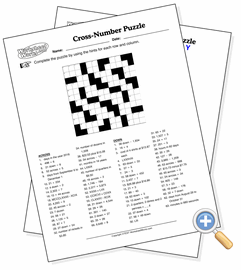Cross-Number PuzzleUse basic math skills to solve a crossword-type puzzle

The cross-number puzzle replaces the words in a crossword with numeric digits, and math equations replace the the word hints. The larger the puzzle you select, the larger the maximum size of the numbers that go into the grid, as well as the more difficult the math problems. You can select the kind of math problems to use, so this puzzle can be made to be solvable by students who are only proficient with their multi-digit addition skills.